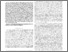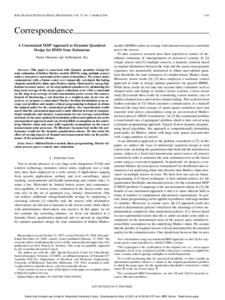# A Constrained MDP Approach to Dynamic Quantizer Design for HMM State Estimation

Ghasemi, Nader and Dey, Subhrakanti (2009) A Constrained MDP Approach to Dynamic Quantizer Design for HMM State Estimation. IEEE Transactions on Signal Processing, 57 (3). pp. 1203-1209. ISSN 1053-587XPreviewmore...Add this article to your Mendeley library

## Abstract

This paper is concerned with dynamic quantizer design for state estimation of hidden Markov models (HMM) using multiple sensors under a sum power constraint at the sensor transmitters. The sensor nodes communicate with a fusion center over temporally correlated flat fading channels modelled by finite state Markov chains. Motivated by energy limitations in sensor nodes, we develop optimal quantizers by minimizing the long term average of the mean square estimation error with a constraint on the long term average of total transmission power across the sensors. Instead of introducing a cost function as a weighted sum of our two objectives, we propose a constrained Markov decision formulation as an average cost problem and employ a linear programming technique to obtain the optimal policy for the constrained problem. Our experimental results assert that the constrained approach is quite efficient in terms of computational complexity and memory requirements for our average cost problem and leads to the same optimal deterministic policies and optimal cost as the unconstrained approach under an irreducibility assumption on the underlying Markov chain and some mild regularity assumptions on the sensor measurement noise processes. We illustrate via numerical studies the performance results for the dynamic quantization scheme. We also study the effect of varying degrees of channel and measurement noise on the performance of the proposed scheme.

Item Type: Article Hidden Markov models; linear programming; Markov decision process; power control; state estimation; Faculty of Science and Engineering > Electronic EngineeringFaculty of Science and Engineering > Research Institutes > Hamilton Institute 14423 https://doi.org/10.1109/TSP.2008.2010381 Subhrakanti Dey 11 May 2021 14:42 IEEE Transactions on Signal Processing Institute of Electrical and Electronics Engineers YesItem control page